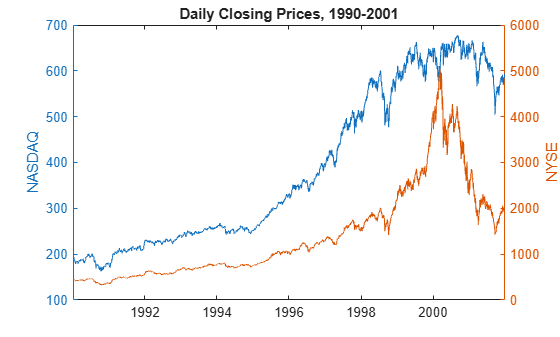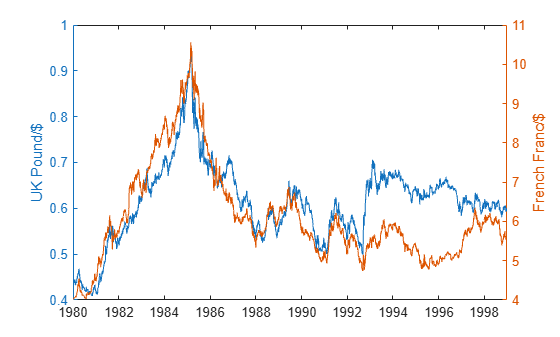# crosscorr

Sample cross-correlation

## Syntax

``[xcf,lags] = crosscorr(y1,y2)``
``XCFTbl = crosscorr(Tbl)``
``````[___,bounds] = crosscorr(___)``````
``[___] = crosscorr(___,Name=Value)``
``crosscorr(___)``
``crosscorr(ax,___)``
``````[___,h] = crosscorr(___)``````

## Description

example

````[xcf,lags] = crosscorr(y1,y2)` returns the sample cross-correlation function (XCF) `xcf` and associated lags `lags` between the univariate time series `y1` and `y2`.```

example

````XCFTbl = crosscorr(Tbl)` returns the table `XCFTbl` containing variables for the sample XCF and associated lags of the last two variables in the input table or timetable `Tbl`. To select different variables in `Tbl`, for which to compute the XCF, use the `DataVariables` name-value argument.```

example

``````[___,bounds] = crosscorr(___)``` uses any input-argument combination in the previous syntaxes, and returns the output-argument combination for the corresponding input arguments and the approximate upper and lower confidence bounds `bounds` on the XCF.```

example

````[___] = crosscorr(___,Name=Value)` uses additional options specified by one or more name-value arguments. For example, ```crosscorr(Tbl,DataVariables=["RGDP" "CPI"],NumLags=10,NumSTD=1.96)``` returns the sample XCF for lags -10 through 10 of the table variables `"RGDP"` and `"CPI"` in `Tbl` and 95% confidence bounds.```

example

````crosscorr(___)` plots the sample XCF between the input series with confidence bounds.```
````crosscorr(ax,___)` plots on the axes specified by `ax` instead of the current axes (`gca`). `ax` can precede any of the input argument combinations in the previous syntaxes.```
``````[___,h] = crosscorr(___)``` plots the sample XCF between the input series and additionally returns handles to plotted graphics objects. Use elements of `h` to modify properties of the plot after you create it.```

## Examples

collapse all

Compute the XCF between two univariate time series. Input the time series data as numeric vectors.

Load the equity index data `Data_EquityIdx.mat`. The variable `Data` is a 3028-by-2 matrix of daily closing prices from the NASDAQ and NYSE composite indices. Plot the two series.

```load Data_EquityIdx yyaxis left dt = datetime(dates,ConvertFrom="datenum"); plot(dt,Data(:,1)) ylabel("NASDAQ") yyaxis right plot(dt,Data(:,2)) ylabel("NYSE") title("Daily Closing Prices, 1990-2001")```The series exhibit exponential growth.

Compute the returns of each series.

`Ret = price2ret(Data);`

`Ret` is a 3027-by-2 series of returns; it has one less observation than `Data`.

Compute the XCF between the NASDAQ and NYSE returns, and return the associated lags.

```rnasdaq = Ret(:,1); rnyse = Ret(:,2); [xcf,lags] = crosscorr(rnasdaq,rnyse);```

`xcf` and `lags` are 41-by-1 vectors that describe the XCF.

Display several values of the XCF.

```XCF = [xcf lags]; XCF([1:3 20:22 end-2:end],:)```
```ans = 9×2 -0.0108 -20.0000 0.0186 -19.0000 -0.0002 -18.0000 0.0345 -1.0000 0.7080 0 0.0651 1.0000 -0.0461 18.0000 0.0010 19.0000 0.0015 20.0000 ```

The correlation between the current NASDAQ return and the NYSE return from 20 days before is `xcf(1) = -0.0108`. The correlation between the NASDAQ and NYSE returns is `xcf(21) = 0.7080`. The correlation between the NASDAQ return from 20 days ago and the current NYSE return is `xcf(41) = 0.0015`.

Compute the XCF between two univariate time series, which are two variables in a table.

Load the equity index data `Data_EquityIdx.mat`. The variable `DataTable` is a 3028-by-2 table of daily closing prices from the NYSE and NASDAQ composite indices, which are stored in the variables `NYSE` and `NASDAQ`.

```load Data_EquityIdx DataTable.Properties.VariableNames```
```ans = 1x2 cell {'NYSE'} {'NASDAQ'} ```

Compute the returns of the series. Store the results in a new table.

```RetTbl = price2ret(DataTable); head(RetTbl)```
```ans=8×4 table Tick Interval NYSE NASDAQ ____ ________ __________ __________ 2 1 -0.0010106 0.0034122 3 1 -0.0076633 -0.0032816 4 1 -0.0084415 -0.0025501 5 1 0.0035387 0.0010688 6 1 -0.010188 -0.0042382 7 1 -0.0063818 -0.013378 8 1 0.0034295 -0.0040909 9 1 -0.023407 -0.020573 ```

`RetTbl` is a 3027-by-4 table containing the returns of the indices, ticks (days by default), and time intervals between successive prices.

Compute the XCF between the NASDAQ and NYSE return series.

`XCFTbl = crosscorr(RetTbl)`
```XCFTbl=41×2 table Lags XCF ____ ___________ -20 -0.010809 -19 0.018571 -18 -0.00016185 -17 -0.020271 -16 -0.029353 -15 0.00023188 -14 -0.0080616 -13 0.041498 -12 0.078821 -11 -0.013793 -10 0.0076655 -9 0.01763 -8 -0.0011033 -7 -0.011457 -6 -0.016523 -5 -0.046749 ⋮ ```

`crosscorr` returns the results in the table `XCFTbl`, where variables correspond to the XCF (`XCF`) and associated lags `(Lags)`.

By default, `crosscorr` computes the XCF of the two variables in the table. To select variables from an input table, set the `DataVariables` option.

Consider the equity index series in Compute XCF of Table Variable.

Load the NYSE and NASDAQ closing price series in `Data_EquityIdx.mat` and preprocess the series. Compute the XCF and return the XCF confidence bounds.

```load Data_EquityIdx RetTbl = price2ret(DataTable); [XCFTbl,bounds] = crosscorr(RetTbl)```
```XCFTbl=41×2 table Lags XCF ____ ___________ -20 -0.010809 -19 0.018571 -18 -0.00016185 -17 -0.020271 -16 -0.029353 -15 0.00023188 -14 -0.0080616 -13 0.041498 -12 0.078821 -11 -0.013793 -10 0.0076655 -9 0.01763 -8 -0.0011033 -7 -0.011457 -6 -0.016523 -5 -0.046749 ⋮ ```
```bounds = 2×1 0.0364 -0.0364 ```

Assuming the NYSE and NASDAQ return series are uncorrelated, an approximate 95.4% confidence interval on the XCF is (-0.0364, 0.0364).

Generate 100 random variates from a Gaussian distribution with mean 0 and variance 1.

```rng(3); % For reproducibility x = randn(100,1);```

Create a 4-period delayed version of `x`.

`y = lagmatrix(x,4);`

Plot the XCF between `x` and `y`. Because `lagmatrix` prepends lagged series with NaN values and `crosscorr` does not support NaN values, start the series at observation 5.

`crosscorr(x(5:end),y(5:end))`The upper and lower confidence bounds are the horizontal lines in the XCF plot. By design, the `XCF` peaks at lag 4.

Load the currency exchange rates data set `Data_FXRates.mat`. The table `DataTable` contains daily exchange rates of several countries, relative to the US dollar from 1980 through 1998 (with omissions).

```load Data_FXRates.mat dt = datetime(dates,ConvertFrom="datenum");```

Plot the UK pound and French franc exchange rates.

```yyaxis left plot(dt,DataTable.GBP) ylabel("UK Pound/\$") yyaxis right plot(dt,DataTable.FRF) ylabel("French Franc/\$")```The series appear to be correlated.

Stabilize all series in the table by computing the first difference.

```DiffDT = varfun(@diff,DataTable); DiffDT.Properties.VariableNames = DataTable.Properties.VariableNames;```

Determine whether lags of one series are associated with the other series by computing the XCF between the daily changes in the UK pound and French franc exchange rates.

```figure crosscorr(DiffDT,DataVariables=["GBP" "FRF"]);```The series have a high contemporaneous correlation, but all other cross-correlations are either insignificant or below 0.1.

Specify the AR(1) model for the first series

`${y}_{1t}=2+0.3{y}_{1t-1}+{\epsilon }_{t},$`

where ${\epsilon }_{t}$ is Gaussian with mean 0 and variance 1.

`MdlY1 = arima(AR=0.3,Constant=2,Variance=1);`

`MdlY1` is a fully specified `arima` object representing the AR(1) model.

Simulate data from the AR(1) model.

```rng(3); % For reproducibility T = 1000; y1 = simulate(MdlY1,T);```

Simulate standard Gaussian variates for the second series; induce correlation at lag 36.

`y2 = [randn(36,1); y1(1:end-36) + randn(T-36,1)*0.1];`

Plot the XCF by using the default settings.

`crosscorr(y1,y2)`All correlations in the plot are within the 2-standard-error confidence bounds. Therefore, none are significant.

Plot the XCF for 60 lags on both sides of lag 0. Specify 3 standard errors for the confidence bounds.

`crosscorr(y1,y2,NumLags=60,NumSTD=3)`The plot shows significant correlations at and around lag 36.

## Input Arguments

collapse all

Univariate time series data, specified as a numeric vector of length T1.

Data Types: `double`

Univariate time series data, specified as a numeric vector of length T2.

Data Types: `double`

Time series data, specified as a table or timetable with T rows. Each row of `Tbl` contains contemporaneous observations of all variables.

Specify the two input series (variables) by using the `DataVariables` argument. The selected variables must be numeric.

Axes on which to plot, specified as an `Axes` object.

By default, `crosscorr` plots to the current axes (`gca`).

Note

Missing observations, specified by `NaN` entries in the input series, result in a `NaN`-valued XCF.

### Name-Value Arguments

Specify optional pairs of arguments as `Name1=Value1,...,NameN=ValueN`, where `Name` is the argument name and `Value` is the corresponding value. Name-value arguments must appear after other arguments, but the order of the pairs does not matter.

Before R2021a, use commas to separate each name and value, and enclose `Name` in quotes.

Example: ```crosscorr(Tbl,DataVariables=["RGDP" "CPI"],NumLags=10,NumSTD=1.96)``` returns the sample XCF for lags -10 through 10 of the table variables `"RGDP"` and `"CPI"` in `Tbl` and 95% confidence bounds.

Number of lags in the sample XCF, specified as a positive integer. `crosscorr` uses lags 0, ±1, ±2, …, ±`NumLags` to compute the sample XCF.

If you supply `y1` and `y2`, the default is min(20, min(T1,T2) – 1)). If you supply `Tbl`, the default is min(20, T – 1).

Example: `crosscorr(y1,y2,NumLags=10)` plots the sample XCF between `y1` and `y2` for lags `–10` through `10`.

Data Types: `double`

Number of standard errors in the confidence bounds, specified as a nonnegative scalar. The confidence bounds are 0 ± `NumSTD*`$\stackrel{^}{\sigma }$, where $\stackrel{^}{\sigma }$ is the estimated standard error of the sample cross-correlation between the input series assuming the series are uncorrelated.

The default yields approximate 95% confidence bounds.

Example: `crosscorr(y1,y2,NumSTD=1.5)` plots the XCF of `y1` and `y2` with confidence bounds `1.5` standard errors away from 0.

Data Types: `double`

Two variables in `Tbl` for which `crosscorr` computes the XCF, specified as a string vector or cell vector of character vectors containing two variable names in `Tbl.Properties.VariableNames`, or an integer or logical vector representing the indices of two names. The selected variables must be numeric.

Example: `DataVariables=["GDP" "CPI"]`

Example: `DataVariables=[true true false false]` or `DataVariables=[1 2]` selects the first and second table variables.

Data Types: `double` | `logical` | `char` | `string`

## Output Arguments

collapse all

Sample XCF between the input time series, returned as a numeric vector of length `2*NumLags` + `1`.

The elements of `xcf` correspond to the elements of `lags`. The center element is the lag 0 cross-correlation. `crosscorr` returns `xcf` only when you supply the inputs `y1` and `y2`.

XCF lags, returned as a numeric vector with elements `(-NumLags):NumLags` having the same orientation as `y1`. `crosscorr` returns `lags` only when you supply the inputs `y1` and `y2`.

Sample XCF, returned as a table with variables for the outputs `xcf` and `lags`. `crosscorr` returns `XCFTbl` only when you supply the input `Tbl`.

Approximate upper and lower XCF confidence bounds assuming the input series are uncorrelated, returned as a two-element numeric vector. The `NumSTD` option specifies the number of standard errors from 0 in the confidence bounds.

Handles to plotted graphics objects, returned as a graphics array. `h` contains unique plot identifiers, which you can use to query or modify properties of the plot.

collapse all

### Cross-Correlation Function

The cross-correlation function (XCF) measures the similarity between a time series and lagged versions of another time series as a function of the lag.

Consider the time series y1,t and y2,t and lags k = 0, ±1, ±2, …. For data pairs (y1,1,y2,1), (y1,2,y2,2), …, (y1,T,y2,T), an estimate of the lag k cross-covariance is

`${c}_{{y}_{1}{y}_{2}}\left(k\right)=\left\{\begin{array}{c}\frac{1}{T}\sum _{t=1}^{T-k}\left({y}_{1,t}-{\overline{y}}_{1}\right)\left({y}_{2,t+k}-{\overline{y}}_{2}\right);\text{\hspace{0.17em}}k=0,1,2,\dots \\ \frac{1}{T}\sum _{t=1}^{T+k}\left({y}_{2,t}-{\overline{y}}_{2}\right)\left({y}_{1,t-k}-{\overline{y}}_{1}\right);\text{\hspace{0.17em}}k=0,-1,-2,\dots \end{array},$`

where ${\overline{y}}_{1}$ and ${\overline{y}}_{2}$ are the sample means of the series.

The sample standard deviations of the series are:

• ${s}_{{y}_{1}}=\sqrt{{c}_{{y}_{1}{y}_{1}}\left(0\right)},$ where ${c}_{{y}_{1}{y}_{1}}\left(0\right)=Var\left({y}_{1}\right).$

• ${s}_{{y}_{2}}=\sqrt{{c}_{{y}_{2}{y}_{2}}\left(0\right)},$ where ${c}_{{y}_{2}{y}_{2}}\left(0\right)=Var\left({y}_{2}\right).$

An estimate of the cross-correlation is

`${r}_{{y}_{1}{y}_{2}}\left(k\right)=\frac{{c}_{{y}_{1}{y}_{2}}\left(k\right)}{{s}_{{y}_{1}}{s}_{{y}_{2}}};\text{\hspace{0.17em}}k=0,±1,±2,\dots \text{.}$`

## Algorithms

• If `y1` and `y2` have different lengths, `crosscorr` appends enough zeros to the end of the shorter vector to make both vectors the same size.

• `crosscorr` uses a Fourier transform (`fft`) to compute the XCF in the frequency domain, and then `crosscorr` converts back to the time domain using an inverse Fourier transform (`ifft`).

• `NaN` values in the input series result in `NaN` values in the output XCF. Unlike `autocorr` and `parcorr`, `crosscorr` does not treat `NaN` values as missing completely at random. Whereas `autocorr` and `parcorr` compute coefficients in the time domain, `crosscorr` uses `fft` and `ifft` to compute coefficients in the frequency domain. Therefore, missing data treatments follow `fft` and `ifft` defaults.

• `crosscorr` plots the XCF when you do not request any output or when you request the fourth output.

 Box, George E. P., Gwilym M. Jenkins, and Gregory C. Reinsel. Time Series Analysis: Forecasting and Control. 3rd ed. Englewood Cliffs, NJ: Prentice Hall, 1994.